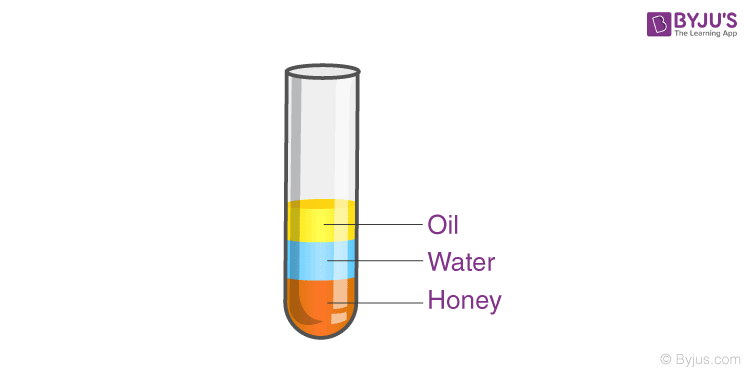# Elementary Idea of Relative Density

In this article, we will understand what is relative density, calculations related to the relative density, and density of various substances. Let us start with the definition of density.

## What Is Density?

Density is the amount of mass in a unit volume of matter; every substance has a different density, to understand the idea of density, let’s conduct an experiment; we will need a tall glass cup, honey, water, coconut oil, and food colouring,

Step1: Pour a one-quarter cup of honey,

Step2: Pour a one-quarter cup of coloured water gently on top of the honey.

Step3: pour a one-quarter cup of coconut oil on top of the colored water.Notice how the different liquids from different layers, why is it so? The different substance has a different density, which means for the same volume different substances weigh differently, as they weigh differently heavier substances tend to settle at the bottom, like honey and lighter material like oil tend to float at the top.

## What Is Relative Density?

The difference between the specific gravity and density is that at room temperature and pressure, 1gram per 1 cubic cm is the density of water this density is treated as a standard and the density of any other material (usual liquids) is calculated relative to this is called relative density or specific gravity.

Hence, specific gravity is the ratio of the mass of a substance to that of a reference substance, let’s consider the density of honey is approx. 1.42 grams/cm3, so its specific gravity would be 1.42/1 = 1.42. Notice that specific gravity is a ratio, therefore specific gravity does not have a unit, and hence specific gravity is a dimensionless physical quantity.

The specific gravity of a substance will let us know if it will float or sink, it gives us the idea about relative mass or relative density. If the specific gravity of a substance is below 1 then it will float and if it is greater than 1 it will sink.

Let’s extend our experiment further for more liquids, this time, we will use several liquids with different specific gravities, use the table given below for reference.

 Material Density(gram/cm3) Rubbing Alcohol 0.79 Lamp Oil 0.8 Baby Oil 0.83 Water 1.0 Milk 1.03 Liquid Soap 1.06 Corn Syrup 1.33 Maple Syrup 1.37 Honey 1.42

Now your assignment would be to calculate the relative density of all these materials with respect to rubbing alcohol and not with water.

### Archimedes Principle

Archimedes principle states that if a body is submerged partially or fully inside a fluid, the body will experience a force that will be equal to the weight of the fluid displaced. This principle is used to determine the purity of gold and other metals, designing of ships, and in lactometers which are used to determine the quality of milk.

## The video about specific gravity## Frequently Asked Questions – FAQs

Q1

### Why does relative density have no unit?

Relative density is the ratio of two similar quantities, so it does not have a unit.
Q2

### Is the relative density of a material constant?

The relative density of any substance is directly relative to its reference. The relative density of a substance relative to oil will be different from the relative density with respect to water.
Q3

### What is the difference between density and relative density?

Density is defined as the ratio of the mass and the volume of a body. On the other hand, relative density is defined as the ratio between the density of a body and the density of some other reference body.
Q4

### What is the common unit of density?

Grams per cubic centimetre.
Q5

### At what temperature, the density of water is maximum?

At 4°C, water has the maximum density.

## Watch the video and solve complete NCERT exercise questions in the chapter Gravitation Class 9To know more about Archimedes principle, specific gravity, and other related concepts by visiting BYJU’S.

Test your Knowledge on Relative Density